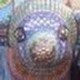# The Unapologetic Mathematician

## A Family of Nontrivial Homology Classes (part 3)

Sorry this didn’t go up as scheduled last week.

We must now show that the forms we’ve defined are closed, and thus that they indeed will define homology classes. That is,$d\omega=0$. We must also show that they cannot be exact, and thus that the homology classes they define are nontrivial.

We defined$\omega$ to be the pullback$\pi^*\hat{\omega}$ of the form$\hat{\omega}$ on the sphere. Thus we can calculate$\displaystyle d\omega=d\pi^*\hat{\omega}=\pi^*d\hat{\omega}=\pi^*0=0$

which follows since$\hat{\omega}$ is an$n$-form on the$n$-dimensional sphere, and so no nontrivial$n+1$-form exists on$S^n$ to be equal to$d\hat{\omega}$.

As for exactness: if$\omega=d\eta$ for some$n-1$-form$\eta$, then Stokes’ theorem tells us that$\displaystyle\int\limits_{S^n}\omega=\int\limits_{S^n}d\eta=\int\limits_{\partial S^n}\eta=\int\limits_\varnothing\eta=0$

Since the sphere has an empty boundary.

But it’s also possible to calculate this integral directly.\displaystyle\begin{aligned}\int\limits_{S^n}\omega&=\int\limits_{S^n}\pi^*\hat{\omega}\\&=\int\limits_{\pi(S^n)}\hat{\omega}\\&=\int\limits_{S^n}\iota_P(du^0\wedge\dots\wedge du^n)\end{aligned}

Now, if$f:U^n\to S^n$ is any parameterization of the sphere, we set$u^i=f^i(x)$ and calculate\displaystyle\begin{aligned}\int\limits_{S^n}\omega&=\int\limits_{S^n}\iota_P(du^0\wedge\dots\wedge du^n)\\&=\int\limits_{U^n}f^*\left(\iota_P(du^0\wedge\dots\wedge du^n)\right)\\&=\int\limits_{U^n}\left[du^0\wedge\dots\wedge du^n\right]\left(P(f(x)),f_{*x}\frac{\partial}{\partial x^1},\dots,f_{*x}\frac{\partial}{\partial x^n}\right)\\&=\int\limits_{U^n}\left[du^0\wedge\dots\wedge du^n\right]\left(u^{i_0}\frac{\partial}{\partial u^{i_0}},\frac{\partial u^{i_1}}{\partial x^1}\frac{\partial}{\partial u^{i_1}},\dots,\frac{\partial u^{i_n}}{\partial x^n}\frac{\partial}{\partial u^{i_n}}\right)\end{aligned}

We extend$f$ to depend on a new variable$x_0$ which will vary in some small neighborhood of$1$ by defining$\tilde{f}(x_0,x)=x_0f(x)$, so that we have$\displaystyle\frac{\partial u^i}{\partial x^0}=u^i$

and so we can continue:\displaystyle\begin{aligned}\int\limits_{S^n}\omega&=\int\limits_{U^n}\left[du^0\wedge\dots\wedge du^n\right]\left(\frac{\partial u^{i_0}}{\partial x^0}\frac{\partial}{\partial u^{i_0}},\frac{\partial u^{i_1}}{\partial x^1}\frac{\partial}{\partial u^{i_1}},\dots,\frac{\partial u^{i_n}}{\partial x^n}\frac{\partial}{\partial u^{i_n}}\right)\\&=\int\limits_{U^n}\det\left(\frac{\partial u^i}{\partial x^j}\right)\left[du^0\wedge\dots\wedge du^n\right]\left(\frac{\partial}{\partial u^0},\dots,\frac{\partial}{\partial u^n}\right)\\&=\int\limits_{U^n}\det\left(\frac{\partial u^i}{\partial x^j}\right)\bigg\vert_{x^0=1}\,d(x^1,\dots,x^n)\end{aligned}

Now, what is this integral calculating? The first column of the determinant is the vector$f(x)$ which, as the position vector of a point on the sphere, has unit length and points perpendicularly to its surface. The other columns form a basis of the tangent space to the sphere at$f(x)$. At any given point, then, we can change the basis so that the upper-left entry in the matrix is$1$ and all other entries in its row and column are zero. The determinant is thus the determinant of the rest of the matrix, which is the Jacobian determinant of the parameterization, and its integral is thus the volume of the image — the volume of the sphere.

Now, we may not have a formula handy for the$n$-dimensional volume of the$n$-sphere, but it’s certainly not zero! Therefore the closed form$\omega$ cannot have been exact, and so it must define a nontrivial homology class, just as asserted.

December 27, 2011 - Posted by | Differential Topology, Topology

1. i couldn’t get anything of it as the base of all this isn’t clear to me!Comment by harrins | December 29, 2011 | Reply

2. Another terrific series! Cohomology isn’t nearly as scary as it sounds.

I’m curious about what you said in (part 1), though, that these “provide models for every possible way a nontrivial homology class can arise” — could you elaborate on that (or point me to where I could learn more)?Comment by Joe English | January 3, 2012 | Reply

3. It’s hard to be rigorous at this point, but the basic idea is that each of these describes a field that can exist around a certain kind of “hole”. For instance, if you can draw (something diffeomorphic to) a sphere around your hole, but that sphere can’t be drawn tight, then you’re looking at something like the three-dimensional version of this construction. If you can draw a circle around the hole that can’t be drawn tight, then you’re looking at the two-dimensional version. This goes for, say, tubular holes bored of a three-dimensional block, because you can draw a loop around them — inside the block — that can’t be drawn tight.

Anyway, given any manifold and a closed form on the manifold, you draw loops of various dimensions around all the holes. If the form is exact, then the integrals around all these loops must be zero; nonzero results are the “obstruction” to the form being exact. But now for each dimension we have a model of a closed-but-not-exact form that just fails to be defined “inside a hole”.

So set up a copy of that model form in your space, with the singularity inside the hole. Scale the model form until the integral around your “test loop” is exactly the same as the integral you got from your original form. Subtract the scaled model form from the original form, and presto — you’ve resolved one obstruction. Do this for all the loops, and the resulting form must be exact. So your original form differs from an exact form by one real number parameter per independent test loop; the only reason wasn’t exact is that it “wrapped around a hole” more or less like one of our models.Comment by John Armstrong | January 3, 2012 | Reply

4. “we may not have a formula handy for the n-dimensional volume of the n-sphere”Comment by John rood | January 5, 2018 | Reply
• Read “we” as authorial, in the context of this one post, not the entire mathematical community. Deriving a single formula is notably more complicated, but not necessary for the result that$\omega$ defines a nontrivial homology class.Comment by John Armstrong | January 5, 2018 | Reply